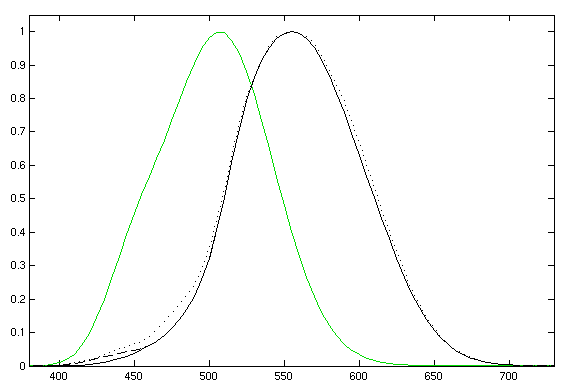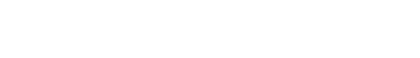# Understanding the Units for LED Output

Selecting the right LED starts with understanding what the datasheet is telling you.

Luminous flux, intensity, brightness, irradiance… When it comes to quantifying LED output, many terms get used in discussions, some with precise meanings and others not. If you’re trying to select an LED for an indication or functional illumination application, you need very specific output. Here, we’ll review the various terms and their meanings so that you can approach data sheets with confidence.

These two classes of optical metrology are related yet distinct from one another. Radiometry refers to measuring light, typically from the infrared to ultraviolet spectral regions. Photometry refers to the measurement of light as perceived by the human eye. The eye does not respond to all wavelengths consistently, so photometry only encompasses the visible waveband and values are scaled by the physiological sensitivity (photopic response) of the eye to a given wavelength (see Figure 1). Radiometric measurements represent real physical value of the characteristic of a source, while photometric measurements represent perceived, or scaled value of a characteristic with respect to the human eye.Figure 1: Plots show the responsivity of the human eye as a function of wavelength in daylight (black curve) and darkness (green curve).

• Radiant flux, also called optical power, is the radiant energy per unit time that is emitted, transmitted, reflected, or received by an object in all directions. It’s expressed in units of watts (W).
• Radiant intensity is the optical power emitted per unit solid angle. It’s an expression of the amount of optical power emitted in a particular direction and is measured in watts per steradian (W/sr).
• Radiance is the optical power emitted by a source per square meter per solid angle. It can be thought of as the area of the sphere subtended by the solid angle that is projected down to a flat surface. It’s measured in units of watts per square meter steradian (W/m2sr).
• Irradiance is the optical power incident on a surface per unit area, measured in units of watts per square meter (W/m2).

Radiometric measurements provide a means of characterizing optical output for instrumentation. When humans are involved, output should be expressed in photometric terms.

## Photometric quantities

Photometric quantities are of greatest importance to LED users. They are weighted by the physiological response of the human eye. Each of the radiometric measurement types has an analogous value in photometry. The photometric quantities consist of the luminous flux, the luminous intensity, the luminance, and the illuminance. They are defined and used as follows:

• Luminous flux, or luminous power, is the perceived amount of light emitted by a source per unit time across one steradian. Basically, it characterizes how much light a source emits in all directions, useful for understanding how effectively a bulb can illuminate a room. It’s measured in units of lumens (lm), or candela steradian (cd⋅sr). This is the quantity frequently shown on the packages of LED bulbs for general lighting applications.
• Luminous intensity is a spectrally weighted measure of how much luminous power a source emits per unit solid angle – how much light a source emits in a specific direction, which is what we really care about when we are using light for a human-machine interface. Luminous intensity is measured in units of lumens per steradian (lm/sr) or candelas (cd), the SI base unit of light metrology. This is the quantity most commonly shown on data sheets for LEDs for indication lighting and functional illumination. It’s typically presented both numerically and graphically (see Figure 2).
• Luminance is the luminous power per unit solid angle. It’s analogous to the radiometric unit of radiance and is measured in candelas per square meter cd/m2 (lm/(sr⋅m2).

Illuminance is the luminous power incident on a surface per unit area. Essentially, it quantifies how much the source illuminates a surface and is measured in terms of Lux (lx), or lumens per square meter (lm/m2).Figure 2: Plot shows relative luminous intensity as a function of peak wavelength for Bivar’s SM0807BC red/green surface mount LED. (Source: Bivar)

## Converting from radiometric units (RUs) to photometric units (PUs)

We convert between radiant flux in watts and luminous flux in lumens using the following expression:where 683 is the conversion factor between 1 W and 1 lm at the peak wavelength for human optical response of 555 nm and V (l) is the photopic response of the human eye.

 Quantity Radiometric Photometric Power Radiant flux, in units of W Luminous flux, in units of Lumen (lm) = cd·sr Power per unit solid angle Radiant intensity, in units of W/sr Luminous intensity, in units of Candela (cd) Power per unit area per unit solid angle Radiance, in units of W/m2·sr Luminance, in units of cd/m2 = lm/m2·sr = nit Power per unit area Irradiance, in units of W/m2 Illuminance, in units of Lux (lx) = cd·sr/m2 = lm/m2

In summary, if a vendor talks about the brightness or intensity of their LED, ask them to express the quantity in proper photometric units to enable you to compare across data sheets. If you have any questions, reach out to your Bivar representative. We’ll be happy to clear up any confusion and ensure that your project is a success.